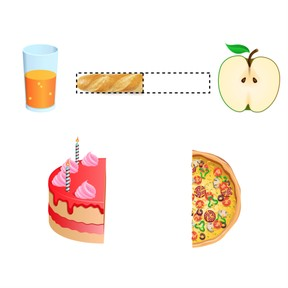Comparing two fractions using the benchmark ½

# Comparing two fractions using the benchmark ½

I can compare two or more fractions using 1//2 as a benchmark.

No account needed.8,000 schools use Gynzy92,000 teachers use Gynzy1,600,000 students use Gynzy

## General

Students will become familiar with the term benchmark. When shown a picture of a full gumball machine, and told that equals 200, students can deduce that 1//2 of that is 100. They will also be introduced to how much of a pointer finger equals an inch, and how that benchmark can be used to quickly estimate a measurement that is about an inch.

## Standards

CCSS.Math.Content.4.NF.A.2

## Learning objective

Students will be able to compare two fractions using the benchmark 1//2.

## Introduction

Students are given a word problem about splitting a candy bar and who gets more. The fraction bar tool helps them to find the answer. They are reminded that although fraction bars are helpful, they aren’t always available to use.

## Instruction

This lesson begins with a brief review of what is a half using various pictures. The students pick out depictions of half. The lesson then continues with a review of the doubles facts of addition to remember the halves of even numbers. To practice, the students will complete a drag-and-drop activity picking out the fractions that mean half. Moving on, there is a discussion about finding the half of an odd number, with another comparison activity using the fraction bar tools. Following this is the strategy to use 1//2 to compare two fractions, followed by practice comparing with the greater than, less than, or equal to signs. The lesson concludes with two word problems comparing fractions.

## Quiz

Students respond to ten multiple-choice and true/false questions.

## Closing

Students will make a flashcard comparing two fractions using the 1//2 benchmark. The answer on the back will also include a drawing of fraction bars to validate their answer.

## Instruction materials

- cardstock cut into flashcards
- something to color with
- ruler

### The online teaching platform for interactive whiteboards and displays in schools

• Save time building lessons

• Manage the classroom more efficiently

• Increase student engagement